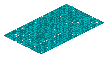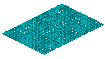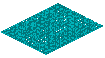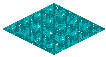LoD1 Test Case 1 (Geometry: lod1MultiSurface)

Test 1

Building length: 6 meter variation +/- 2 meter

Building width: 8 meter variation +/- 2 meter

Building height: 50 meter variation +/- 20 meter

Offset between the building x=y: 10 meter

yearOfConstruction: between 1980 and 2006

Number of Buildings 10 x 10 = 100 20 x 20 = 400 50 x 50 = 2500 100 x 100 = 10000
Screen Shot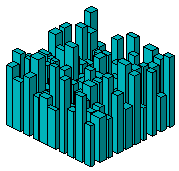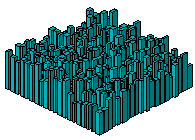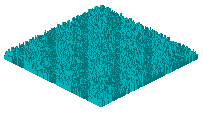Test 2

Building length: 6 meter

Building width: 8 meter

Building height: 5 meter variation +/- 2 meter

Offset between the building x=y: 10 meter

building class: between 1000 and 1180

yearOfConstruction: between 1900 and 2000

yearOfDemolition: between 2020 and 2100

roofType: between 1000 and 1130

measuredHeight: from building height

storeysAboveGround: calculated by measuredHeight/storeyHeightsAboveGround

storeysBelowGround: 0

storeyHeightsAboveGround: between 2 and 3 meter

storeyHeightsBelowGround: 0 meter

Number of Buildings 100 x 10 = 1000 100 x 20 = 2000 100 x 30 = 3000 100 x 40 = 4000 100 x 50 = 5000
Screen Shot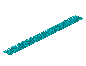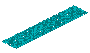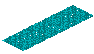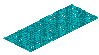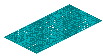Screen Shot